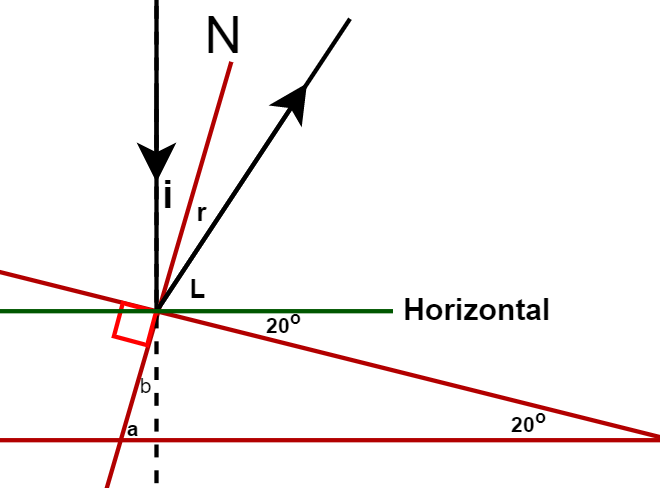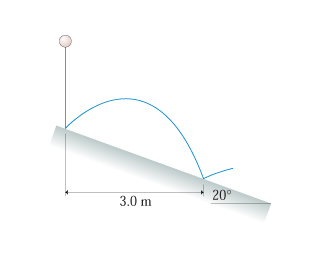# Problem: A rubber ball is dropped onto a ramp that is tilted at 20 , as shown in the figure. A bouncing ball obeys the "law of reflection," which says that the ball leaves the surface at the same angle it approached the surface. The balls next bounce is 3.0 { m m} to the right of its first bounce.What is the balls rebound speed on its first bounce?

###### FREE Expert Solution

In this problem, we are given a ball that is launched from the surface through reflection.

Uniform accelerated motion (UAM) equations, a.k.a. "kinematics equations":

2D vectors Direction/Components:

$\overline{)\mathbf{|}\stackrel{\mathbf{⇀}}{\mathbf{v}}\mathbf{|}{\mathbf{=}}\sqrt{{{\mathbf{v}}_{\mathbf{x}}}^{\mathbf{2}}\mathbf{+}{{\mathbf{v}}_{\mathbf{y}}}^{\mathbf{2}}}}$

$\overline{){\mathbf{tan}}{\mathbit{\theta }}{\mathbf{=}}\frac{{\mathbit{v}}_{\mathbit{y}}}{{\mathbit{v}}_{\mathbit{x}}}}$

We'll determine the launch angle from the laws of reflection.

Law of reflection: Angle of incidence is equal to the angle of reflection.84% (79 ratings)###### Problem Details

A rubber ball is dropped onto a ramp that is tilted at 20 , as shown in the figure. A bouncing ball obeys the "law of reflection," which says that the ball leaves the surface at the same angle it approached the surface. The balls next bounce is 3.0 to the right of its first bounce.

What is the balls rebound speed on its first bounce?